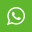## Material Content for PROGRAMMING AND PROBLEM SOLVING THROUGH C LANGUAGE JULY 2014

1. Each question below gives a multiple choice of answers. Choose the most appropriate one and enter in the “OMR” answer sheet supplied with the question paper, following instructions therein. (1x10)

1.1 Which of the following function declaration need not have a return statement in its body?

A) int a(char *s)

B) void b(int a[], int n)

C) float *c()

D) short d(long x)

1.2 Identify the correct sequence of steps to run a program

1.3 What will be the output of the following code segment?

char *p = "Structured Programming";

printf("%s", p + 5);

A) Structured Programming

B) tured Programming

C) ctured Programming

D) There will be no output as there is a syntax error in code

1.4 Which of the following is an incorrect syntax?

A) void outerFn() {

...

int innerFn(float f) {

...

}

}

B) struct outer {

...

struct inner {

...

}I;

};

C) if (i < 10) {

…

if (x == y) {

...

}

...

}

D) for (i = 0; i < 10; i++) {

...

for (j = 0; j < 10; j++) {

...

}

}

1.5 For the following definitions

char a[] = "Hello World!";

int i;

Which of the following loop will print the output as Hello World!

A) for (i = 0; a[i] != '/0'; i++)

printf("%s", a[i]);

B) for (i = 0; a[i] != '/0'; i++)

printf("%c", a[i]);

C) for (i = 0; a[i] != '\0'; i++)

printf("%s", a[i]);

D) for (i = 0; a[i] != '\0'; i++)

printf("%c", a[i]);

1.6 Consider the statement given below:

int a = {1, 2, 3, 4, 5}, *p = a;

Which printf statement will print the value of fourth element of the array?

A) printf("%d ", *(p + 3));

B) printf("%d", p);

C) printf("%d ", a + 3);

D) printf("%d ", *a + 3);

1.7 What will be the output of the following code segment?

int x = 24, y = 39, z = 45;

z = x + y;

y = z - y;

x = z - y;

printf("\n%d %d %d", x, y, z);

A) 24 39 63

B) 39 24 63

C) 24 39 45

D) 39 24 45

1.8 Which of the following is the correct way to define a two dimensional array?

A) int a[ ];

B) int b[2, 4];

C) int c[ ];

D) int d[ ] [ 4] = {{1, 3, 5, 7}, {2, 4, 6, 8}};

1.9 What will be the output of the following code segment if Hello there is given as input?

char a;

scanf("%s", a);

printf("%s", a);

A) Hello there

B) Hello

C) "Hello there"

D) "Hello"

1.10 What will be the output of the following code segment?

void fn() {

static int i = 10;

printf("%d ", ++i);

}

main() {

fn();

fn();

}

A) 10 10

B) 11 11

C) 11 12

D) 12 12

2. Each statement below is either TRUE or FALSE. Choose the most appropriate one and ENTER in the “OMR” answer sheet supplied with the question paper, following instructions therein. (1x10)

2.1 A printf statement can be used to display output on different lines.

2.2 # is used to insert comments in the program.

2.3 The statement fopen("data.txt", "w+") will open a file named data.txt for both reading and writing.

2.4 Structures can have bit fields.

2.5 If no storage class is mentioned for a variable defined in a function then it is by default auto.

2.6 It is necessary to have default case in a switch statement.

2.7 A continue statement must be enclosed in a loop.

2.8 The names of parameters in a function definition and its declaration must be same.

2.9 sizeof() is a function used to determine the amount of memory occupied by a variable.

2.10 The address of the variable is passed to a function in call by reference.

3. Match words and phrases in column X with the closest related meaning/ word(s)/phrase(s) in column Y. Enter your selection in the “OMR” answer sheet supplied with the question paper, following instructions therein. (1x10)

 question option 3.1 Terminates program A. union 3.2 Calls itself B. Typecasting 3.3 Dynamic memory allocation C. strcmp() 3.4 Space for one member only D. "%[^\n]" 3.5 Explicit conversion E. fseek() 3.6 p -> member_name F. exit() 3.7 File pointer movement G. Recursive functions 3.8 String comparison H. Array 3.9 Unary operator I. ++ 3.10 Reading line with scanf() J. malloc() K. (*p).member_name L. Structure M. fabs()

4. Each statement below has a blank space to fit one of the word(s) or phrase(s) in the list below. Enter your choice in the “OMR” answer sheet supplied with the question paper, following instructions therein. (1x10)

4.1 ________ is used to club variables of different data types as a unit.

4.2 It is necessary to have a pointer to the next node in ________.

4.3 ________ loop is executed atleast once.

4.4 If there is no test condition in a for loop then it becomes a(n) ________ loop.

4.5 Avoid the use of ________ statement in the program.

4.6 An operator which can change the value of a variable on the right hand side of an expression is ________.

4.7 If a function is defined as fn(char a){ … }, then its return type is ________.

4.8 Format code in printf to print exactly 10 characters is ________.

4.9 A ________ variable stores the address of another variable.

4.10 ________ can be used to write in a file.